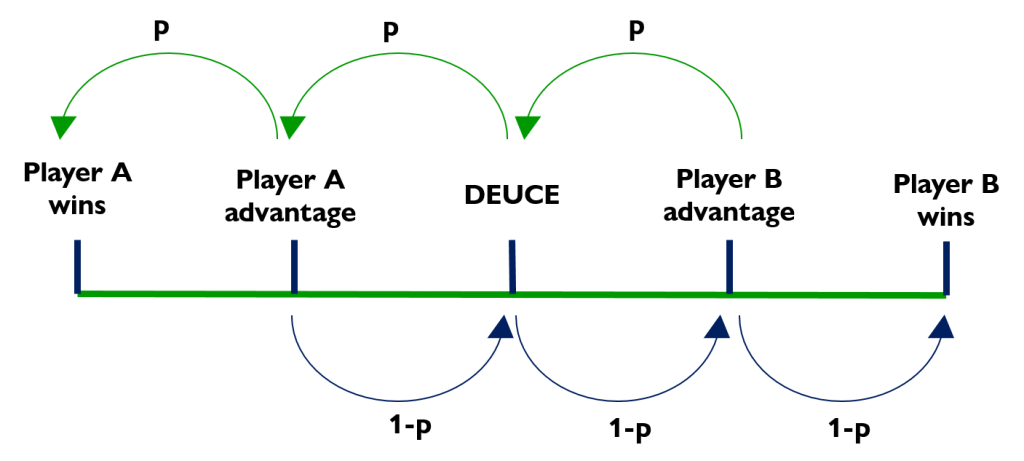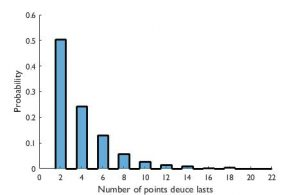# Tennis maths: how long should a deuce point last?

Using Markov chains to calculate some interesting tennis stats!Summer is upon us, and we’re almost halfway through Wimbledon, so there’s no better time to look at some tennis maths!

Tennis actually has a variety of interesting mathematical behaviour. The game has quite a strange scoring system, which can lead to some interesting curiosities. For example, you could win a match at Wimbledon having only won 35% of the points. In 2010, there was a famous set at Wimbledon which  ended 70-68. Fields medallist Timothy Gowers investigated, and found you should only expect such an occurrence to happen once every 200 years!  And last year, we used aerodynamics to investigate why a tennis serve curves so much.

In this post, I’m going to look at how some simple applications of probability can be used to give us some interesting statistics. For those not completely acquainted with the rules of tennis, there is a position called deuce. At this point, if one player win a point, they go to advantage, and if they win the next point they win the game. However if at advantage, they lose that point, the game returns to deuce. (The idea is that each game has to be won by two clear points.)

Whenever I am playing tennis, and I get to deuce, very often it seems like the game gets stuck with no winners for a long time. Every time I get to advantage, I feel like I crumble under the pressure, and can never win that point. But when my opponent is at advantage, and I’m on the verge of defeat, I always seem to be able to pull off some incredible recovery shots, and we keep returning to deuce.

But is this actually true, or is this just a story I’ve made up in my head? Do we expect a typical deuce point to last for a long time?

### What’s the probability of winning a deuce point?

Well this is actually a reasonably simple mathematical model to describe. There are five states the game can be in: Deuce, Player A advantage, Player B advantage, Player A win, Player B win. We can think of this as a random walk on the following line:Now in order to model the situation, we will need to make an initial working assumption. Let us assume that Player A has a probability $p$ that they will win each point, where $0\leq p\leq 1$, and let us assume this is the case no matter whether they are at deuce or up or down an advantage.  Therefore, since probabilities have to add up to one, Player B has a probability $1-p$ that they win each point.

Mathematicians would call this a random walk with two absorbing states. One can think of it being like a walk on the above line, with each step taking you from one state to an adjacent one. Two of the states are called absorbing, because as soon as the system gets to either end of the line, the game ends. This system is memoryless: since it only matters where on the line you are at any particular time, it doesn’t care about what has happened previously in the game. This makes the system a particularly simple example of a statistical process known as a Markov chain.

Before we look at the expected time for one player to win, let us look at the probability Player A wins, given that we start at deuce. We can do this with some simple conditional probability. Firstly, we will need to introduce some notation. Let us call

• $P_D$- the probability Player A wins assuming the game is at deuce.
• $P_{Ba}$- the probability Player A wins assuming the game is at Advantage for Player B.
• $P_{Aa}$- the probability Player A wins assuming the game is at Advantage for Player A.

A deuce point always starts at deuce, so our goal is to calculate $P_D$. We use the common notation $P(A|B)$ to mean the probability event A happens given event B. Now if we are at deuce, there are two outcomes for the next point. Player A could either win the point, with probability $p$, or lose it, with probability $1-p$. Thus we can write the following equation

$$P_D = P(\text{Player A wins} | \text{Player A wins first shot}) P(\text{Player A wins first shot}) + P(\text{Player A wins} | \text{Player A loses first shot}) P( \text{Player A loses first shot})$$

or, in our notation,

$$P_D = p P_{Aa}+ (1-p) P_{Ba}.$$

So, we have found $P_D$. But we don’t know what $P_{Ba}$ or $P_{Aa}$ are! But using conditional probability in exactly the same way, we can derive equations for $P_{Aa}$ and $P_{Ba}$. We find

$$P_{Aa} = p + (1-p) P_D$$

$$P_{Ba} = p P_D$$

We end up with a system of three equations, for three unknown quantities $P_{D}, P_{Ba}, P_{Aa}$, and we can simply use simultaneous equations to find the answers. So, we find that if I have a probability $p$ of winning each point, I have the following probability for winning the game if I’m at deuce

$$P_D= \frac{p^2}{2 p^2-2 p+1}.$$

An important sanity check is to put $p=1/2$ into the above equation. If both players have an equal chance of winning each point, then we expect them to have an equal chance of winning the game at deuce. And indeed we do find $P_D=1/2$ in this case. What is interesting about this is that the equation is nonlinear in the probability. If I am a slightly better player than you, and thus have a slightly better chance of winning each point, I have a much greater chance of winning a game that is at deuce. We can see this more clearly if we plot $P_D$ against $p$ in a graph:This behaviour is replicated throughout the rest of tennis’ scoring system. For a game to be close, it requires players to be extraordinarily similar in ability.  At the highest level, a small marginal gain in the probability of winning a point can make the difference between obliterating an opponent or being humiliatingly defeated. So the fact that so many tennis matches between professionals are so close suggests that the players are extraordinarily close to each other in ability.

I solved all the above using explicit simultaneous equations. But I could have used matrix methods instead, and for a more complicated system that would have been necessary. Using matrices allows you to unleash the full power of Markov chains, which are particularly important in areas such statistics and finance.

So that’s the probabilities dealt with. What about my original question, how long should a deuce point typically last?

## How long should a deuce point last?

Actually, we can perform a very similar analysis that we just did. Let us denote the average number of points to be played, (or the expected number of points to be played), given we are in a certain state as follows:

• $E_D(t)$- the expected number of points of the game assuming the game is at deuce.
• $E_{Ba}(t)$- the expected number of points of the game assuming the game is at Advantage for Player B.
• $E_{Aa}(t)$- the expected number of points of the game assuming the game is at Advantage for Player A.

We can now do a super similar analysis as we did in the probabilities, obtaining three simultaneous equations for our three unknowns. We find that the expected number of points of the game at deuce point is

$$E_D(t)=\frac{2}{2 p^2-2 p+1}$$

This is maximum when $p=1/2$ (as you’d expect, the game should last longer if both players are equal), and in this case the expected length of a deuce point is $4$ points: 1 advantage, back to deuce, another advantage and then a winner.

Ok, but my deuce points seem to last a lot longer than $4$ points, I feel like I’ve often had games that have lasted for over $12$ points. The expectation alone though cannot tell us whether this is likely though. We need to find out what the distribution of the game time is. It may turn out that the distribution has quite a fat tail, in which case it might be likely to have long lasting deuce points.A little bit of thought gives us the probability that a game at deuce will last a further $2n$ points. Think about the first two points. One player wins the first point, and there is a probability of $1/2$ of the deuce ending, or a probability of $1/2$ of getting back to deuce, being back where we started. So the probability should halve each time, and we get the result

$$P(\text{deuce lasting }2n \text{ points}) =\frac{1}{2^n}$$

But I’m sure my deuce points last longer than this pattern suggests. Although perhaps this could just be confirmation bias on my part, I remember the long games because they are noteworthy, and forget all the times they ended really quickly. But nonetheless, let’s look at how the game length changes if we alter the model. Let us say every time I get to Advantage, I find the pressure so much that my probability of winning the point halves, to 0.25. However, if my opponent is at Advantage, they crumble under the pressure, and thus my determination not to loose kicks in, and my probability of winning increases to 0.75.

So I ran some simulations modelling this, running the deuce point $10,000$ times, and I found that we should expect the following distribution:As we can see, this causes games to last a lot longer in general, with short games occurring much more infrequently. Sadly I have not collected a detailed record of every tennis game in my life up until now (although from now on I will), so I cannot actually test my hypothesis and I certainly don’t think the psychological effect is as big as the one shown in the above graph! But it would be interesting to observe what form the distribution length of deuce points in professional tennis takes. If anyone would be interested in looking into the actual data on this do get in touch!Matt is a PhD student at UCL, working in the fields of general relativity and cosmology.
ucl.ac.uk/~ucahawr    + More articles by Matthew